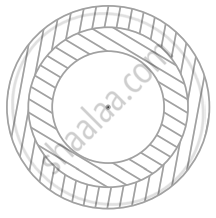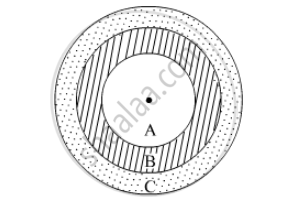# An archery target has three regions formed by three concentric circles as shown in figure. If the diameters of the concentric circles are in the ratios 1 : 2 : 3 , then find the ratio of the areas - Mathematics

Sum

An archery target has three regions formed by three concentric circles as shown in figure. If the diameters of the concentric circles are in the ratios 1 : 2 : 3 , then find the ratio of the areas of three regions.#### SolutionLet the three regions be A, B and C.

The diameters are in the ratio 1 : 2 : 3.

Let the diameters be 1x, 2x and 3x

Then the radius will be x/2, (2x)/2  and (3x)/2

Area of region A = pi r_A^2 = pi(x/2)^2 = pix^2/4

Area of region B = pi r _B^2-pi r _A^2 = pi(x)^2-pi(x/2)^2 = (3pi(x)^2)/4

Area of region C = pi r_C^2-pi r_B^2-pi r_A^2 = pi((3x)/2)^2-pi(x)^2-pi(x/2)^2 = pi((3x)/2)^2-(3pix^2)/4 = (5pix^2)/4

Thus, ratio of the areas of regions A, B and C  will be

pix^2/4 : (3pi(x)^2)/4 : (5pi x^2)/4

⇒ 1 : 3 : 5

Concept: Area of Circle
Is there an error in this question or solution?

#### APPEARS IN

NCERT Mathematics Exemplar Class 10
Chapter 11 Area Related To Circles
Exercise 11.4 | Q 13 | Page 134
RD Sharma Class 10 Maths
Chapter 13 Areas Related to Circles
Exercise 13.1 | Q 20 | Page 12

Share